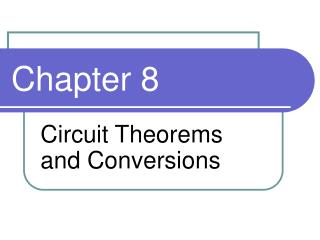DownloadDownload PresentationChapter 8

Chapter 8

Download PresentationChapter 8

- - - - - - - - - - - - - - - - - - - - - - - - - - - E N D - - - - - - - - - - - - - - - - - - - - - - - - - - -
Presentation Transcript

1. Chapter 8 Circuit Theorems and Conversions

2. Objectives • Apply the superposition theorem to circuit analysis • Apply Thevenin’s theorem to simplify a circuit for analysis • Apply Norton’s theorem to simplify a circuit • Apply the maximum power transfer theorem

3. Voltage Source • The voltage source ideally provides constant voltage to a load even when the load resistance varies. • In reality, no voltage source is ideal. • Voltage sources have some inherent internal resistance (represented by RS) • When a load is applied to the source, not all of the voltage appears across RL, since some of the voltage is dropped across RS • If RS is very small compared to RL • The source approaches ideal because almost all of the source voltage, VS, appears across the larger resistance, RL

4. Current Source • The ideal constant current source has an infinitely large internal parallel resistance. • Transistors act basically as current sources (the equivalent model of a transistor does contain a current source). • In a practical current source, some of the current, IS, is through RS, and part is through RL. • If RS >> RL, the current source approaches ideal.

5. Converting a Voltage Source to a Current Source The voltage source can be converted to an equivalent current source by: IS = VS/RS

6. Converting a Current Source to a Voltage Source The current source can be converted to an equivalent voltage source by: VS = ISRS

7. Find Vs

8. Superposition Theorem • Some circuits require more than one voltage or current source. • The superposition theorem is a way to determine currents and voltages in a circuit that has multiple sources by considering one source at a time. • General Statement of Superposition Theorem • The current in any given branch of a multiple-source circuit can be found by determining the currents in that particular branch produced by each source acting alone, with all other sources replaced by their internal resistances. The total current in the branch is the algebraic sum of the individual source currents in that branch.

9. Example

10. Find the Current Through R2

11. Find the Current Through R3

12. Thevenin’s Theorem • Thevenin’s theorem provides a method for simplifying a circuit to a standard equivalent form. • The Thevenin equivalent voltage (VTH) is the open circuit (no-load) voltage between two terminals in a circuit. • The Thevenin equivalent resistance (RTH) is the total resistance appearing between two terminals in a given circuit with all sources replaced by their internal resistances.

13. Thevenin Equivalent of a Circuit

14. Find VTH and RTH across A & B

15. Norton’s Theorem • Norton’s theorem provides a method for simplifying a circuit to a standard equivalent form. • The Norton equivalent current (IN) is the current between two terminals in a circuit. • The Norton equivalent resistance (RN) is the total resistance appearing between two terminals in a given circuit with all sources replaced by their internal resistances.

16. Summary of Norton’s Theorem • Connect IN and RN in parallel to produce the complete Norton equivalent for the original circuit (as shown to the right).

17. Find the Norton equivalent

18. Maximum Power Transfer • When a source is connected to a load, maximum power is delivered to the load when the load resistance is equal to the internal source resistance. • The source resistance, RS, of a circuit is the equivalent resistance as viewed from the output terminals using Thevenin’s theorem. • A typical application of the maximum power transfer theorem is in audio systems, where the speaker resistance must be matched to the audio power amplifier in order to obtain maximum output.

19. Show the load power curve (PRL) Assume: RL = ½ RS RL = RS RL =2 RS

20. Summary